# 绘制整系数多项式复数根的分布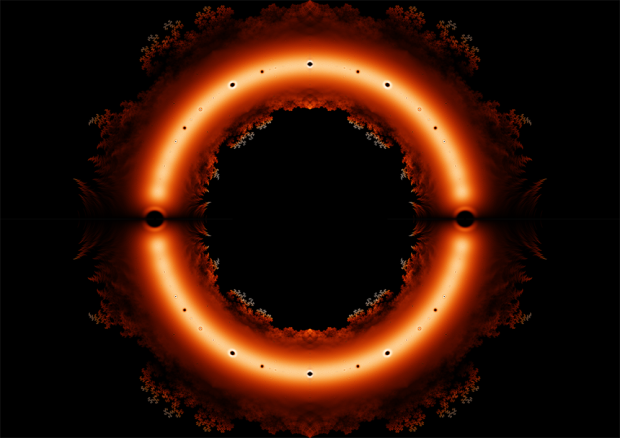• 如何求解多项式的根
• 图上的颜色按照什么绘制

/*程序功能：求解所有指定次数的、系数为-1、1的多项式，并把复数解保存到r[0-7].data中

#include <stdio.h>
#include <gsl/gsl_poly.h> //GNU science library 多项式
#include <sys/wait.h>
#include <unistd.h>

#define deg 24 //多项式次数

int main(){
int i,j,p,total;
double a[deg+1],z[deg*2]; //a:多项式系数 z:求解结果
FILE *fp;
char fn;
a[deg]=1.0; //减少一半运算

for(p=0;p<8;p++){
if(fork()==0){
sprintf(fn,"r%d.data",p);
fp=fopen(fn,"wb");
for(i=0;i<3;i++) //该进程的三个确定系数
a[deg-1-i]=(p&(1<<i))?1.0:-1.0;
total=(1<<(deg-3));
for(i=0;i<total;i++){
for(j=0;j<deg-3;j++)
a[j]=(i&(1<<j))?1.0:-1.0;
gsl_poly_complex_workspace *w=gsl_poly_complex_workspace_alloc(deg+1);
gsl_poly_complex_solve(a,deg+1,w,z); //求解
gsl_poly_complex_workspace_free(w);
fwrite(z,sizeof(double),deg*2,fp);

if(i%10000==0)printf("Process%d : %.2f%%\n",p,i*100.0/total);
}
printf("Process%d : Completed\n",p);
exit(0);
}
}
while(wait(NULL)>0); //等待其他进程
return 0;
}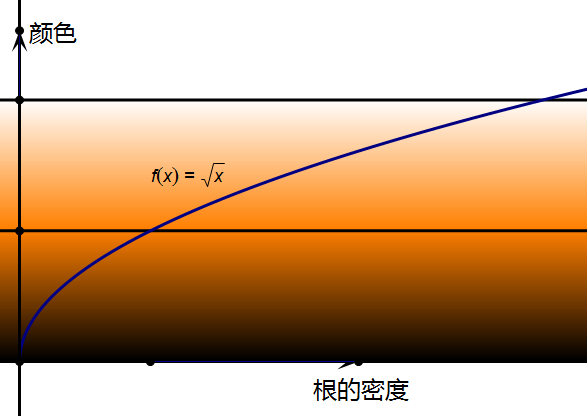/*程序功能：根据r[0-7].data中的复数数据，生成密度图*/
#include <stdio.h>
#include <stdlib.h>
#include <math.h>

#define DIM 1024 //图片分辨率 DIM*DIM
#define RANGE 2.0 //绘制范围 x,y∈[-RANGE,RANGE]
#define B 12.0 //亮度微调参数，越大越暗
#define BUFFSIZE ((1<<24)*24/8*2) //读文件缓冲区大小，24次多项式约800M

double *data; //读文件缓冲区
int (*den)[DIM]; //像素点范围内根的个数
unsigned char (*bitmap)[DIM]; //用于生成位图

int total=0,max;

void countroot(int cnt){ //按照像素区域对方程的根计数
double x,y;
int i,px,py;
for(i=0;i<cnt;i++){
x=data[i*2];
y=data[i*2+1];
px=DIM/2*x/RANGE+DIM/2; //计算对应的像素坐标
py=DIM/2*y/RANGE+DIM/2;
if(px>0&&px<DIM&&py>0&&py<DIM)
den[px][py]++;
}
total+=cnt; //总点数计数
}

void colorfunc(int d,unsigned char *color){ //根密度与颜色的映射函数
int t;
t=(long long)255*sqrt((double)d/max);
if(t<256){ //黑至橙渐变
color=t;
color=t/2;
color=0;
}else if(t<512){ //橙至白
color=255;
color=128+(t-256)/2;
color=t-256;
}else{ //白
color=color=color=255;
}
}

void genpic(){ //根据密度数据生成位图
int i,j;
printf("Generating pic...\n");
max=B*total/DIM/DIM; //颜色255对应的根密度
for(j=0;j<DIM;j++)
for(i=0;i<DIM;i++)
colorfunc(den[i][j],bitmap[j][i]);
}

void savefile(){ //保存文件
char fn;
sprintf(fn,"roots_%d.ppm",DIM);
FILE *fp=fopen(fn,"wb");
printf("Saving to %s...\n",fn);
fprintf(fp,"P6\n%d %d\n255\n",DIM,DIM);
fwrite(bitmap,1,DIM*DIM*3,fp);
fclose(fp);
printf("Completed!\n");
}

int main(){
int p,cnt;
char fn;
FILE *fp;
data=malloc(BUFFSIZE*sizeof(double));
den=malloc(DIM*DIM*sizeof(int));
bitmap=malloc(DIM*DIM*3);
if(!data||!den||!bitmap){
printf("Out of memory!\n");
exit(-1);
}
for(p=0;p<8;p++){
sprintf(fn,"r%d.data",p);
fp=fopen(fn,"rb");
fseek(fp,0,SEEK_END); //定位到文件尾
cnt=ftell(fp)/sizeof(double)/2; //根据文件大小计算数据量
fseek(fp,0,SEEK_SET); //定位到文件头
fclose(fp);
printf("Processing : %s\n",fn);
countroot(cnt);
}
genpic();
savefile();
return 0;
}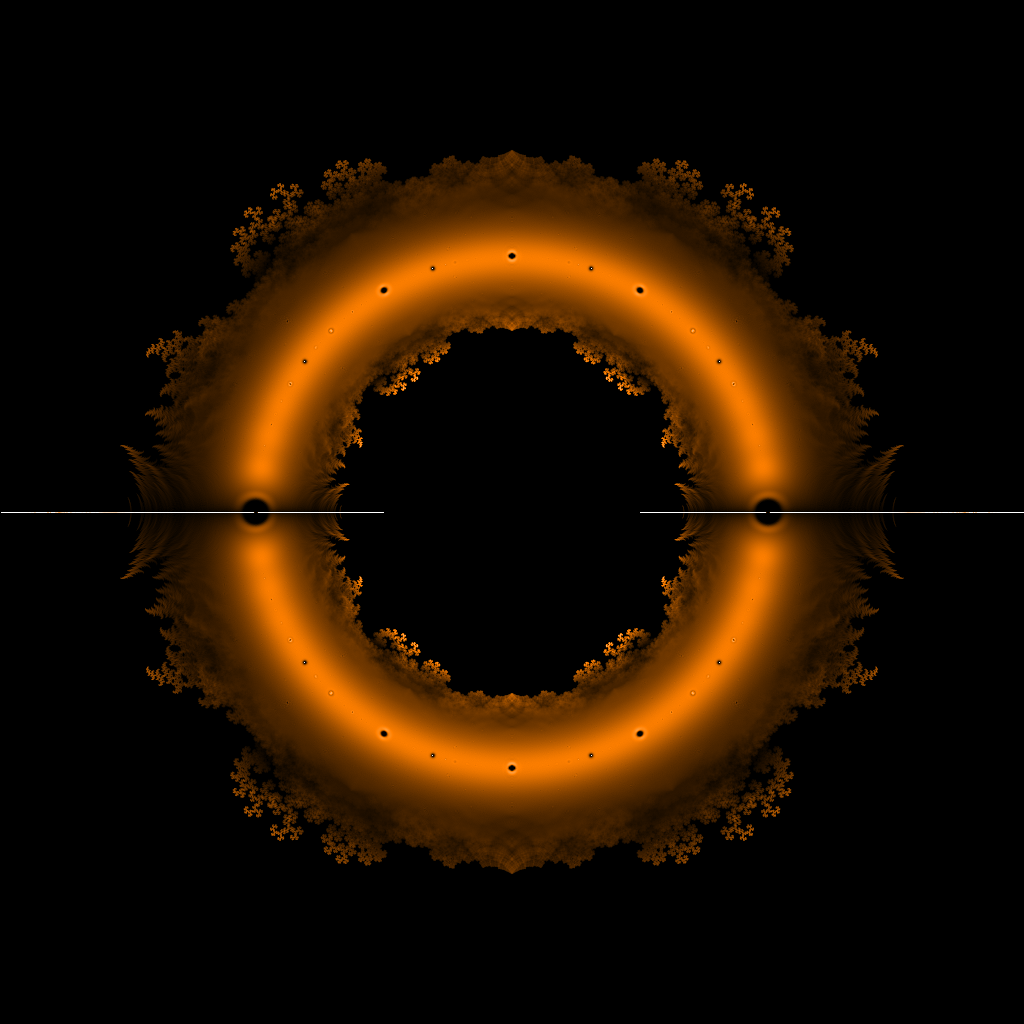gsl: zsolve.c:78: ERROR: root solving qr method failed to converge Default GSL error handler invoked.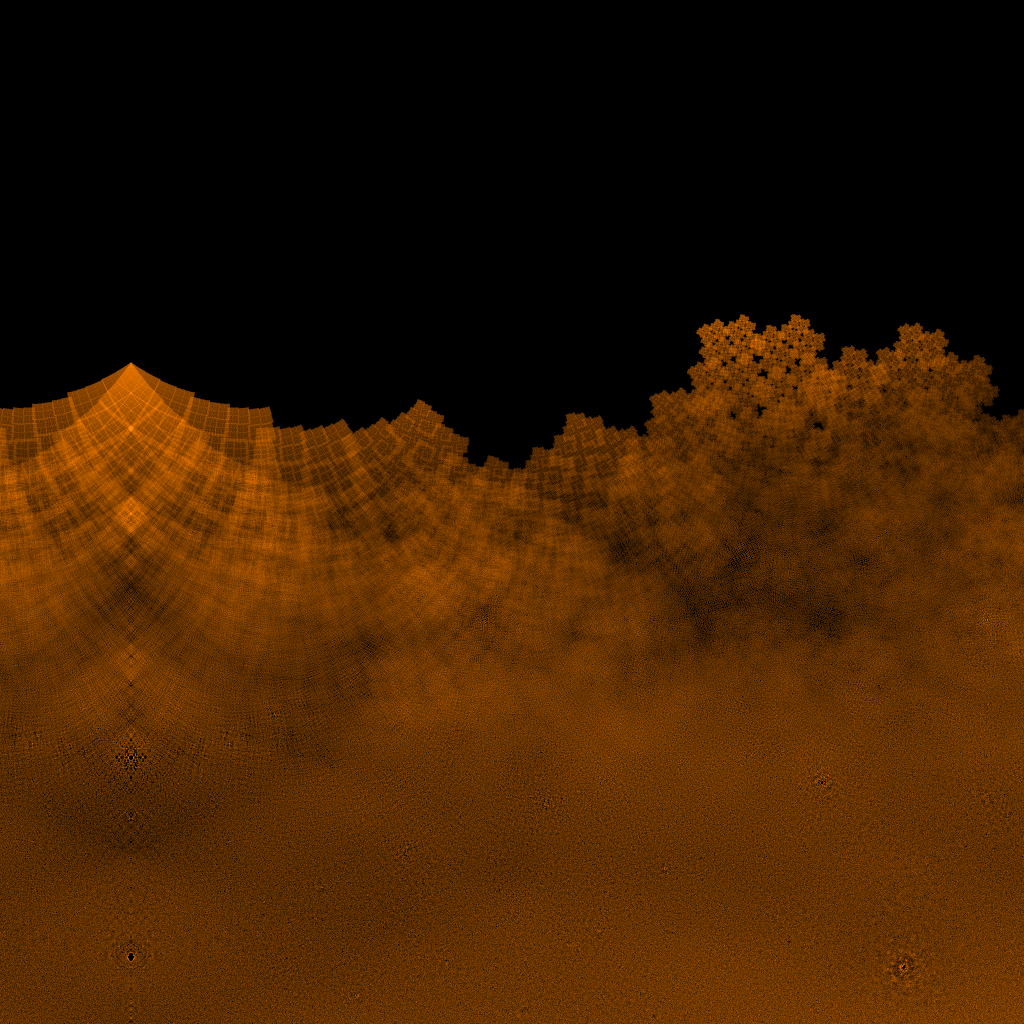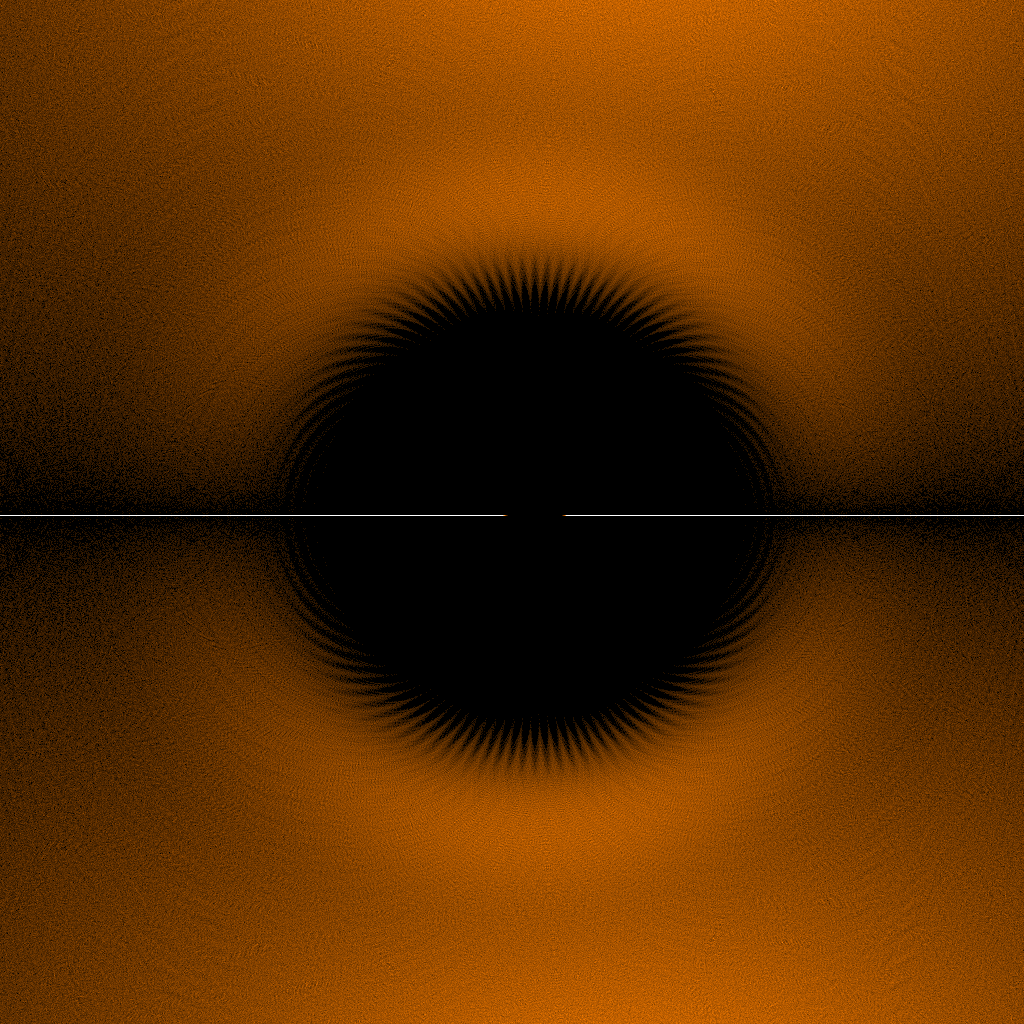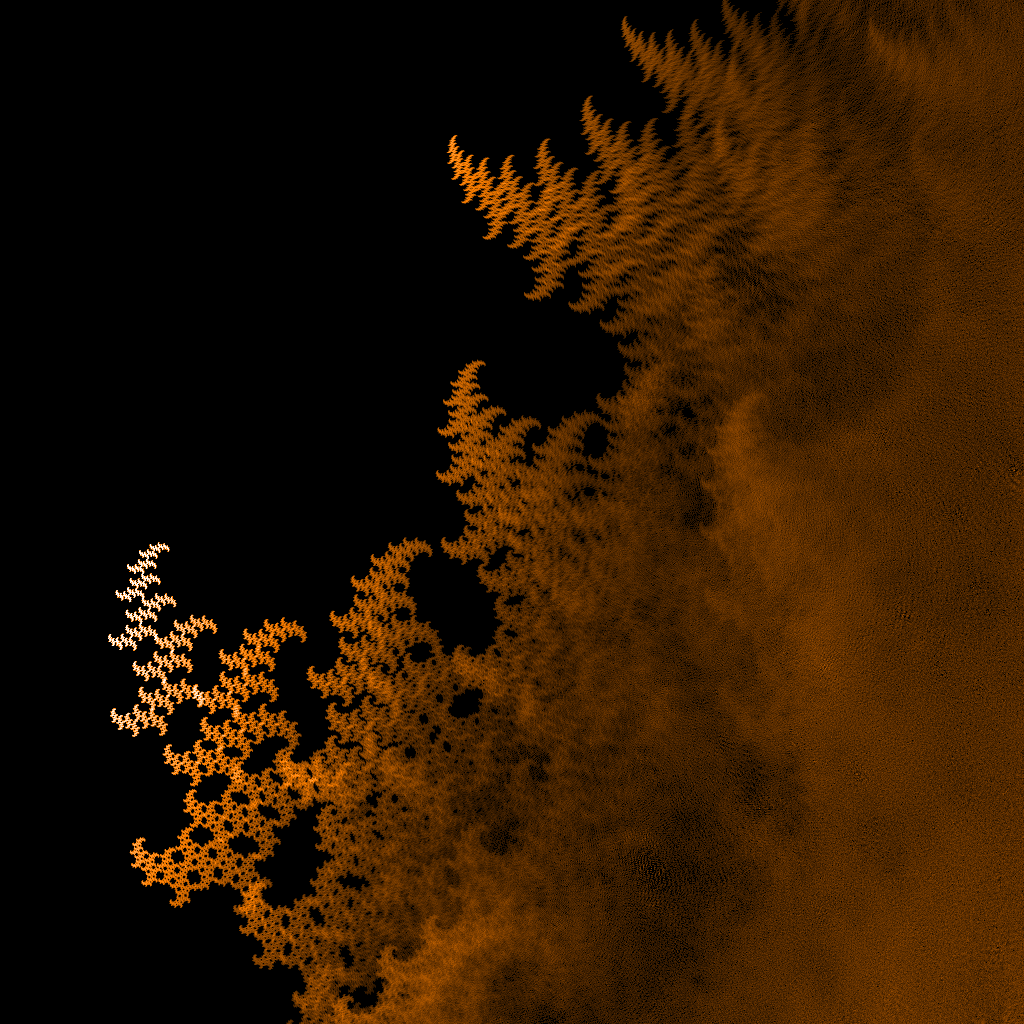## 《绘制整系数多项式复数根的分布》有一个想法

1. Xiangru Lian说道：

Getting patterns in a structured problem is ordinary.. It should not be described as “the Beauty of Math”….